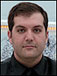# A Hands-on Introduction to R

## OSCON 2013 Speaker Series

R is an open-source statistical computing environment similar to SAS and SPSS that allows for the analysis of data using various techniques like sub-setting, manipulation, visualization and modeling. There are versions that run on Windows, Mac OS X, Linux, and other Unix-compatible operating systems.

To follow along with the examples below, download and install R from your local CRAN mirror found at r-project.org. You’ll also want to place the example CSV into your Documents folder (Windows) or home directory (Mac/Linux).

After installation, open the R application. The R Console will pop-up automatically. This is where R code is processed. To begin writing code, open an editor window (File -> New Script on Windows or File -> New Document on a Mac) and type the following code into your editor:

`1+1`

Place your cursor anywhere on the “1+1” code line, then hit Control-R (in Windows) or Command-Return (in Mac). You’ll notice that your “1+1” code is automatically executed in the R Console. This is the easiest way to run code in R. You can also run R code by typing the code directly into your R Console, but using the editor is much easier.

If you want to refresh your R Console, click anywhere inside of it and hit Control-L (in Windows) or Command-Option-L (in Mac).

Now let’s create a Vector, the simplest possible data structure in R. A Vector is similar to a column of data inside a spreadsheet. We use the `combine` function to do so:

`raysVector <- c(2, 5, 1, 9, 4)`

To view the contents of `raysVector`, just run the line of code above. After running the code shown above, double-click on `raysVector` (in the editor) and then run the code that is automatically highlighted after double-clicking. You will now see the contents of `raysVector` in your R Console.

The object we just created is now stored in memory and we can see this by running the following code:

`ls()`

R is an interpreted language with support for procedural and object-oriented programming. Here we use the `mean` statistical function to calculate the statistical mean of `raysVector`:

`mean(raysVector)`

Getting help on the `mean` function is easy using:

`?mean`

We can create a simple plot of `raysVector` using:

`barplot(raysVector, col = "red")`

Importing CSV files is simple too:

`data <- read.csv("raysData3.csv", na.strings = "")`

We can subset the CSV data in many different ways. Here are two different methods that do the same thing:

```data[ 1:2, 2:4 ]
data[ 1:2, c("age", "weight", "height") ]```

There are many ways to transform your data in R. Here’s a method that doubles everyone’s age:

`dataT <- transform( data, age = age * 2 )`

The `apply` function allows us to apply a standard or custom function without loops. Here we apply the mean function `column-wise` to the first 3 rows of the dataset in order to analyze the age and height columns of the dataset. We will also ignore missing values during the calculation:

`apply( data[1:3, c("age", "height")], 2, mean, na.rm = T )`

Here we build a linear regression model that predicts a person’s weight based on their age and height:

`raysModel <- lm(weight ~ age + height, data = data)`

We can plot our residuals like this:

`plot(raysModel\$residuals, pch = 15, col = "red")`

We can install the Predictive Model Markup Language (PMML) package to quickly deploy our predictive model in a Business Intelligence system without custom SQL:

`install.packages("pmml")`

After running the code above, you may need to select a mirror if this is the first time you are installing an R package on your system. Select any mirror you like.

After installing the `pmml` package, we now load the package into memory – so we can use the package:

`library(pmml)`

Now we export our regression model to PMML format, which is an open-standard format based on XML. The PMML file will export to your working directory after running the following code:

`saveXML(pmml(raysModel, dataset = data), "pmml.xml")`

If you are unsure where your working directory is located, just run this code:

`getwd()`

If you want to learn more about PMML, here is a short video that provides a high-level explanation.

As you can see, it’s pretty easy to get going with R. It allows you to get right to manipulating data and converting it to a usable format is a breeze.